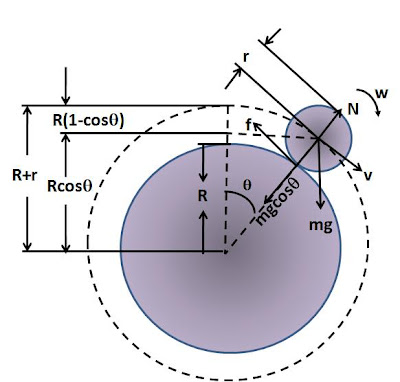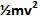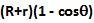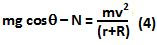Thursday, December 18, 2008

Irodov Problem 1.263Let the tangential velocity of the smaller sphere (its center) be v and its angular velocity about its own center be w. As the sphere rolls down down it looses gravitational potential energy. This loss in potential energy is completely converted into its kinetic energy (since the sphere rolls no energy is lost due to friction). Its kinetic energy is the sum of its translational energy () and its rotational kinetic energy (). When the smaller sphere's CG is at an angle, the CG of the sphere would have come down a height ofas shown in the figure. Since the moment of inertia of the smaller sphere about its axis of rotation iswe haveSince the sphere is rolling, we have,Now let us consider the forces acting on the sphere in the direction normal to the surface of the sphere. There are two forces acting in this direction, i) the component of force of gravityand ii) the normal reaction from the surface of the larger sphere N. The acceleration of the smaller sphere in the normal direction is the centripetal acceleration due to the rotation of its CG about the center of the larger sphere directed inwards towards the center. So we have,When the smaller sphere breaks off of the surface of the larger sphere, the normal reaction N will becomes zero (since there is no contact anymore). Thus, from (2) and (4) and setting N=0 we have,Now substituting (5) in (3) we have,